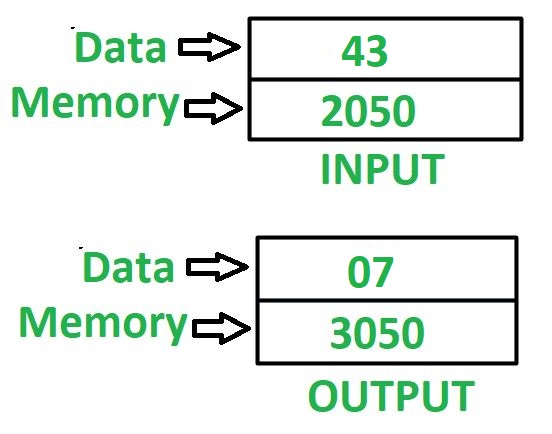# 8085 program to find sum of digits of 8 bit number

• Last Updated : 03 Jun, 2022

Problem: Write an assembly language program in 8085 microprocessor to find the sum of digits of an 8-bit number.

Example:Assumptions: Addresses of input data and output data are 2050 and 3050 respectively.

Algorithm:

1. Load value stored at memory location 2050 in accumulator A
2. Move the value of accumulator A in register B to process 43H.
3. Perform masking of nibbles i.e. do AND operation of accumulator A with OF with help of ANI (Immediate Addressing Mode with AND) instruction. We will get a lower nibble value in accumulator A. Because of 0F, Higher Nibble will be masked and a lower nibble will be obtained as it is.
4. Move the value of accumulator A in register C, Because currently, the Accumulator value is the value of a lower nibble so [C] = Lower nibble value = 3H
5. Move the value of register B in accumulator A, Because the value of the Accumulator is updated hence we will again move the Value of B into Accumulator.
6. Reverse the number which is stored in accumulator A by using RLC instruction 4 times and again do masking of nibbles as done in step 3.
7. Add the value of register C in accumulator A
8. Store the value of A in memory location 3050

(After using 4 Times RLC, we will get reversed of given number because after 4 times, 4 bits will move left and again it will be rotated to MSB to LSB and vice-versa hence we will get reversed number, And after using ANI 0F, we will get Lower nibble values in both the cases. Hence after adding these 2 values using ADD C instruction, we are getting a sum of digits in a given number.)

Program –

Explanation: Registers used A, B, C

1. LDA 2050: loads the content of memory location 2050 in accumulator A
2. MOV B, A: moves the value of accumulator A in register B
3. ANI 0F: performs AND operation in value of accumulator A and 0F
4. MOV C, A: moves the value of accumulator A in register C
5. MOV A, B: moves the value of register B in accumulator A
6. RLC: instruction rotates the value of accumulator A, left by 1 bit. Since it is performed 4 times therefore this will reverse the number i.e swaps the lower order nibble with a higher-order nibble
7. Repeat step 3
8. ADD C: Add the content of the register of C in accumulator A
9. STA 3050: Stores value of A in 3050
10. HLT: Stops executing the program and halts any further execution

ADDITIONAL INSTRUCTION: This task can be done in a 2nd way by using 4 times RRC instruction.

LDA 2050

ANI 0F

MOV C, A

MOV A, B

RRC

RRC                            //4 RRC do same work as 4 RLC. So we can use anyone alternatively.

RRC

RRC

ANI 0F

STA 3050

HLT

END

```Example :
16H in Binary Written as :
0001 0110
RLC 1st Time : 0010 1100  {Carry Flag = 0}
RLC 2nd Time : 0101 1000  {Carry Flag = 0}
RLC 3rd Time : 1011 0000  {Carry Flag = 0}
RLC 4th Time : 0110 0001 { Carry Flag = 1}
Converted Number after 4th RLC : 0110 0001 [61H]
Hence our number is reversed from 16H to 61H.

For Example : 16H in Binary Written as :
0001 0110
RRC 1st Time : 0000 1011  {Carry Flag = 0}
RRC 2nd Time : 1000 0101  {Carry Flag = 1}
RRC 3rd Time : 1100 0010 { Carry Flag = 1}
RRC 4th Time : 0110 0001 { Carry Flag = 0}
Converted Number after 4th RRC : 0110 0001 [61H]
Hence our number is reversed from 16H to 61H.
Hence Instead of 4 RLC, we can also use 4 RRC instructions in our code and
accordingly AND them with 0F in the same way as above.```
My Personal Notes arrow_drop_up
Recommended Articles
Page :